Courses

# Test: Data Interpretation - 1 (2019-2014)

## 20 Questions MCQ Test UPSC Topic Wise Previous Year Questions | Test: Data Interpretation - 1 (2019-2014)

Description
This mock test of Test: Data Interpretation - 1 (2019-2014) for UPSC helps you for every UPSC entrance exam. This contains 20 Multiple Choice Questions for UPSC Test: Data Interpretation - 1 (2019-2014) (mcq) to study with solutions a complete question bank. The solved questions answers in this Test: Data Interpretation - 1 (2019-2014) quiz give you a good mix of easy questions and tough questions. UPSC students definitely take this Test: Data Interpretation - 1 (2019-2014) exercise for a better result in the exam. You can find other Test: Data Interpretation - 1 (2019-2014) extra questions, long questions & short questions for UPSC on EduRev as well by searching above.
QUESTION: 1

### The following three items are based on the graph given below which shows imports of three different types of steel over a period of six months of a year. Study the graph and answer the three items that follow.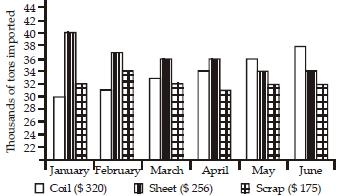The figures in the brackets indicate the average cost per ton over six months period. Q. By how much (measured in thousands of tons) did the import of sheet steel exceed the import of coil steel in the first three months of the year? 

Solution:

Total import of sheet steel in first three months  = 40 + 37 + 36 = 113
Total import of coil steel in first three months = 30 + 31 + 33 = 94
Required value = 113 – 94 = 19.

QUESTION: 2

### The following three items are based on the graph given below which shows imports of three different types of steel over a period of six months of a year. Study the graph and answer the three items that follow.The figures in the brackets indicate the average cost per ton over six months period. Q. What was the approximate total value (in \$) of sheet steel imported over the six months period? [2018

Solution:

Total sheet steel important over six months period = 40 + 37 + 36 + 36 + 34 + 34 = 217
Total value = 217 × 256 = 55552 = 55550

QUESTION: 3

### The following three items are based on the graph given below which shows imports of three different types of steel over a period of six months of a year. Study the graph and answer the three items that follow.The figures in the brackets indicate the average cost per ton over six months period. Q. What was the approximate ratio of sheet steel and scrap steel imports in the first three months of the year? [2018

Solution:

Total sheet steel imported in first three months = 40 + 37 + 36 =113
Total scrap steel imported in first three months = 32 + 34 + 32 = 98
Required ratio = 113 : 98 = 1.2:1

QUESTION: 4

The following graph indicates the composition of our tax revenue for a period of two decades :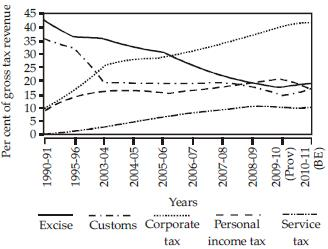With reference to the above graph, which of the following is/are the most logical and rational inference/ inferences that can be made?
1. During the given period, the revenue from Direct Taxes as percentage of gross tax revenue has increased while that of Indirect Taxes decreased.
2. The trend in the revenue from Excise Duty demonstrates that the growth of manufacturing sector has been negative during the given period.
Select the correct answer using the code given below.



Solution:

1. Corporate tax (……) and personal tax (-----) are direct taxes. From graph it is clear that corporate tax is increasing tremendously whereas personal tax increased slightly.
Exercise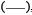customs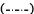and service tax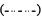are indirect taxes. From the graph, it is clear that exercise and customs decreased tremendously whereas service tax is increased.
Therefore, during the given period, the revenue from direct taxes as percentage of gross tax revenue has increased while that of indirect taxes decreased.
2. From the graph, it is clear that exercise duty is over all decreased tremendeusly. But after 2010, exercise duty increases slightly. This traineds of exercise duty has many reasons. One of then may be decreasing rate of exercise duty to promote manufacturing sector thats why after 2010 exercise duty increases slightly.

QUESTION: 5

The following table gives the GDP growth rate and Teledensity data of different States of a country in a particular year. Study the table and answer the two items that follow.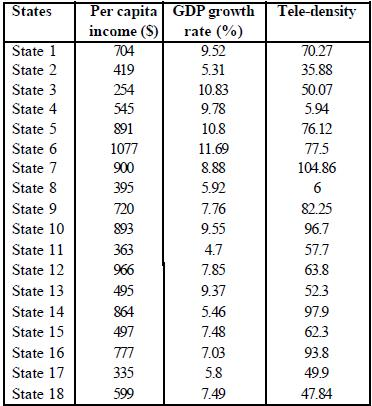Q. With reference to the above table, which of the following is/are the most logical and rational inference/inferences that can be made?
1. Higher per capita income is generally associated with higher Tele-density.
2. Higher GDP growth rate always ensures higher per capita income.
3. Higher GDP growth rate does not necessarily ensure higher Tele-density.
Select the correct answer using the code given below.



Solution:

According to the table tele-density is similar to others

QUESTION: 6

The following table gives the GDP growth rate and Teledensity data of different States of a country in a particular year. Study the table and answer the two items that follow.Q. With reference to the above table, the following assumptions have been made:
1. Nowadays, prosperity of an already high performing State cannot be sustained without making further large investments in its telecom infrastructure.
2. Nowadays, a very high Tele-density is the most essential condition for promoting the business and economic growth in a State.
Which of the above assumptions is/are valid?



Solution:

There are many points related to GDP of state and tele-density is similar to others according to table.

QUESTION: 7

The graph given below indicates the changes in key policy rates made by the Central Bank several times in a year: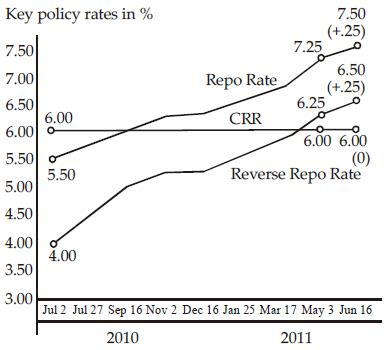Which one of the following can be the most likely reason for the Central Bank for such an action?



Solution:

Repo rate and reverse repo rate is directly related to Anti-inflationary stance

QUESTION: 8

Consider the following graphs. The curves in the graphs indicate different age groups in the populations of two countries A and B over a period of few decades: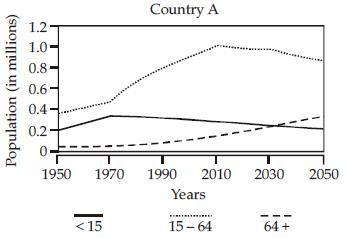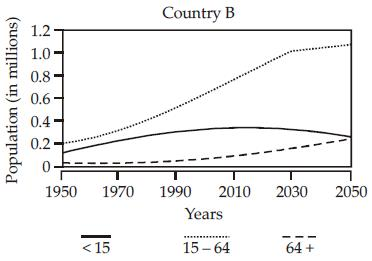With reference to the above graphs, which of the following are the most logical and rational inferences that can be made?
1. Over the last two and a half decades, the dependency ratio for country B has decreased.
2. By the end of next two and a half decades, the dependency ratio of country A will be much less than that of country B.
3. In the next two decades, the work-force relative to its total population will increase in country B compared to country A.
Select the correct answer using the code given below.



Solution:

Dependency Ratio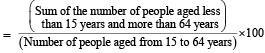1. In country B, over the last two and a half decades (2025 to 2050), the sum of the number of people aged less than 15 years and more than 64 years is slightly increased but number of people aged from 15 to 64 years increased tremendously.  Therefore dependency radio of country B has decreased over the last two and half decades.
2. By the end of next two and a half decades (i.e. 2075), sum of the number of people aged less than 15 years and more than 64 years of country A is more than that of country B where as number of people of aged from 15 to 64 years of country A is less than that of country B.
Hence by the end of next two and a half decades, the dependency ratio of country A will be more than that of country B.
3. In the next two decades (i.e. 2050-2070), graph of workforce (i.e. population of aged from 15 years to 64 years) of country A is going lower and that of country B is going higher.
Therefore, in the next two decades, the work-force relative to its total population will increase in country B as compared to country A.

QUESTION: 9

Consider the following figures A and B: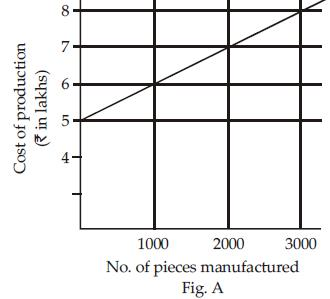The manufacturing cost and projected sales for a product are shown in the above figures A and B respectively. What is the minimum number of pieces that should be manufactured to avoid a loss?



Solution:

Manufacturing cost of 1000 pieces = 600000
Cost of 1 piece when 1000 pieces manufactured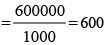Selling price of 1 piece when 1000 pieces manufactured = 400
Manufacturing cost of 2000 pieces = 700000
Cost of 1 piece when 2000 pieces manufactured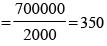Selling price of 1 piece when 2000 pieces manufactured = 350
Hence, required number of pieces = 2000

QUESTION: 10

Consider the following graph in which the birth rate and death rate of a country are given, and answer the two items that follow.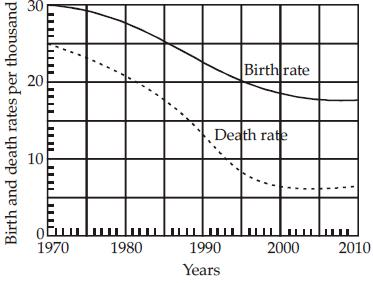Q. Looking at the graph, it can be inferred that from 1990 to 2010



Solution:

According to the graph, death rate is decreased faster than birth rate, therefore population growth rate has increased.

QUESTION: 11

Consider the following graph in which the birth rate and death rate of a country are given, and answer the two items that follow.Q. With reference to the above graph, consider the following statements considering 1970 as base year:
1. Population has stabilized after 35 years.
2. Population growth rate has stabilized after 35 years.
3. Death rate has fallen by 10% in the first 10 years.
4. Birth rate has stabilized after 35 years.
Which of the above are the most logical and rational statements that can be made from the above graph?



Solution:

After 2005 birth rate and population growth has no change.

QUESTION: 12

Consider the following graph: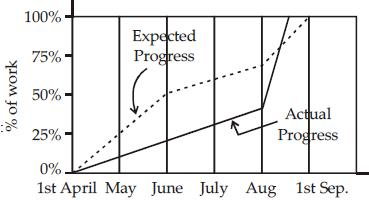Which one of the following statements is not correct with reference to the graph given above?



Solution:

According to the given graph, during mid of the August, actual progress was more than expected progress.

QUESTION: 13

The proportion of expenditure on various items by two families A and B are represented in the following Bar Charts: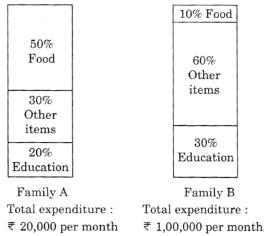From these charts, we can conclude that



Solution:

A spent on food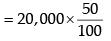=  ₹10000
B spent on food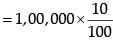= ₹100, 00
So, Both A and B spent same amount on the food.

QUESTION: 14

Year-wise variation of the price of a certain commodity is shown in the following graph: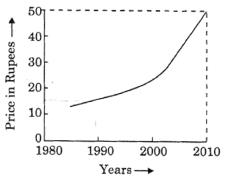The price of the commodity in the year 1990



Solution:

From the graph commodity price are between ₹ 10 and ₹ 20.

QUESTION: 15

The graph below depicts the earnings of A and B over the period 2000 to 2010: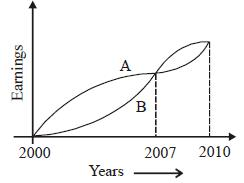From the graph, which one of the following can be concluded?



Solution:

From given years 2000 to 2007
Earnings of A > Earnings of B
So,
Average of A > Average of B
Hence, It can be conducted that the average A earned more than B during this period.

QUESTION: 16

An automobiles owner reduced his monthly petrol consumption when the prices went up. The price consumption relationship is as follows: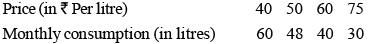If the price goes up to ₹ 80 per litre, his expected consumption (in litres) will be



Solution:

The product of price (in ₹/litre) and the monthly consumption (in litres) in constraint is equal to 2400.
Expected consumption when the price goes up to ₹ 80 per litre = 2400/80 = 30 litres

QUESTION: 17

The following table gives population and total income of a city for four years :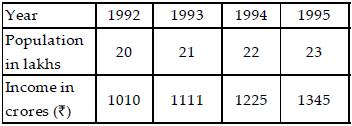Which one of the following statements is correct inrespect of the above data?



Solution:

Rule out options:-
Option (a): - We clearly see that from 1993 to 94, increase in pop is less than 5%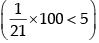Option (b): - from year 1994 to 1995
Increase in income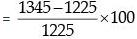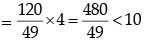∴ Not true
Option (c): per capita income (p.c.i.)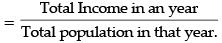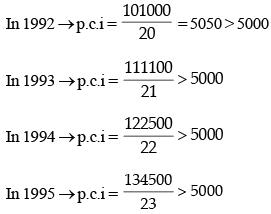Option (c) is correct.

QUESTION: 18

The following table shows the marks obtained by two students in different subjects :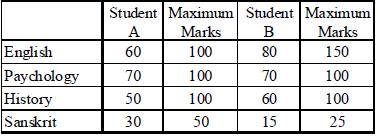The difference in the mean aggregate percentage marks of the students is



Solution:

For A Agg marks = 60 + 70 + 50 + 30 = 210
Agg max marks = 100 + 100 + 100 + 50 = 350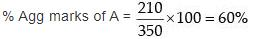For B:
Agg marks = 80 + 70 + 60 + 15 = 225
Agg max marks = 150 + 100 + 100 + 25 = 375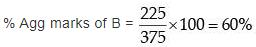Difference in agg percentage = 0
Note: Difference in agg percentage is same as difference in mean agg percentage.

QUESTION: 19

The following graph shows the average profit of two fruit-sellers A and B in thousands (₹) per year from the year 1995 to 2000. Consider the graph and answer the 4 (four) items that follow :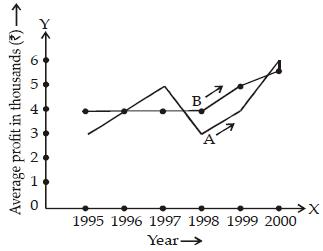Q. In which year is the average profit of A and B same?



Solution:

From the given options, in 1996, the graphs of A & B intersect, which shows, same average profit.

QUESTION: 20

The following graph shows the average profit of two fruit-sellers A and B in thousands (₹) per year from the year 1995 to 2000. Consider the graph and answer the 4 (four) items that follow :Q. What is the difference between the average profit of B and A in the year 1998?



Solution:

From the graph, we observe that the difference between points A & B is more than half of 1000, but less than 1000.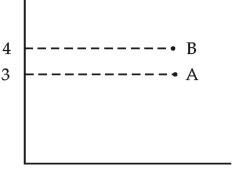∴ 500 < |Difference between A and B| < 1000.
From the option, + 600 satisfies this condition.